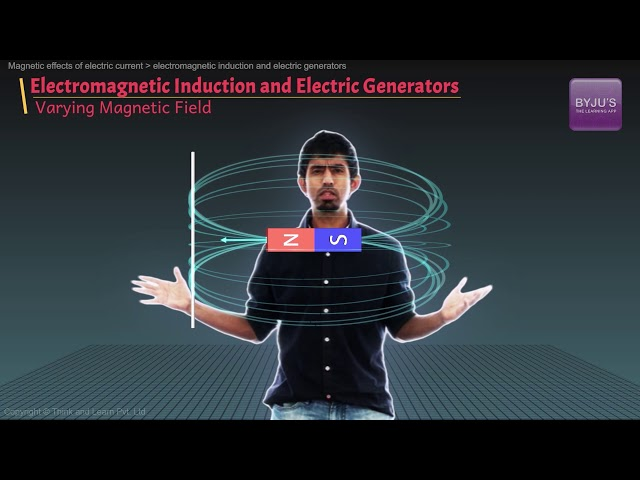# Electromagnetic Induction

## What is Electromagnetic Induction?

Electromagnetic Induction was discovered by Michael Faraday in 1831 and James Clerk Maxwell mathematically described it as Faraday’s law of induction.

Electromagnetic Induction is a current produced because of voltage production (electromotive force) due to a changing magnetic field.

This either happens when a conductor is placed in a moving magnetic field (when using AC power source) or when a conductor is constantly moving in a stationary magnetic field.

Michael Faraday arranged a conducting wire as per the setup given below, attached to a device to measure the voltage across the circuit. When a bar magnet was moved through the coiling, the voltage detector measures voltage in the circuit.Through his experiment, he discovered that there are certain factors that influence this voltage production. They are:

1. Number of Coils: The induced voltage is directly proportional to the number of turns/coils of the wire. Greater the number of turns, greater is voltage produced
2. Changing Magnetic Field: Changing magnetic field affects the induced voltage. This can be done by either moving the magnetic field around the conductor or moving the conductor in the magnetic field.

You may also want to check out these concept related to induction:

## Applications of Electromagnetic Induction

Based on his experiments we now have Faraday’s law according to which the amount of voltage induced in a coil is proportional to the number of turns of the coil and the rate of changing magnetic field.

### Electromagnetic Induction Formula

Mathematically, the induced voltage can be given by the following relation:

 e = N × dΦ / dt

Where,

• e is the induced voltage (in volts)
• N is the number of turns in the coil
• Φ is the magnetic flux – the amount of magnetic field at a surface (in Webbers)
• t is the time (in seconds)

The significance of this discovery is a way of producing electrical energy in a circuit by using magnetic fields and not just batteries. Everyday machines like motors, generators and transformers work on the principle of electromagnetic induction.Did you know that the principle of electromagnetic induction is also involved in the working of a credit card strip when you swipe it across the card machine? How do you think this works? [Hint: The strip is magnetic]

Stay tuned with BYJU’S to learn more Physics concepts with the help of interactive video lessons.